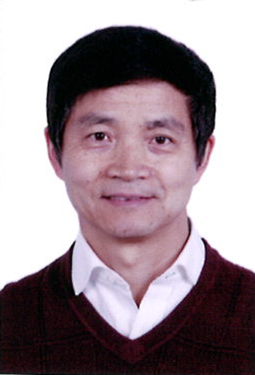Applied and Computational MathematicsDivision research focus

The research of the applied mathematics lab covers a broad spectrum of basic research topics of applied and computational mathematics such as numerical analysis and numerical partial differential equations, to interdisciplinary research topics of mathematical modeling and scientific computing such as uncertainty quantification and flow control. Much of the ongoing and planned research places emphasis on the development of fundamental mathematical concepts and innovative numerical algorithms towards the mathematical analysis, multiscale and stochastic modeling and simulations of problems in fluid and solid mechanics, atomic and soft matter physics, biological and materials sciences, data and image analysis, and other application domains.

Division Research Areas
• ·  Numerical analysis and numerical partial differential equations

1) General theory for numerical PDE such as finite difference, finite element, spectral methods.

2) Numerical methods and analysis for various problems such as oscillatory PDEs, Integral equations, singularly perturbed problems, problems in unbounded domains, mesh generation and optimization, fractional and nonlocal models.

• ·  Mathematical modeling and scientific computing

1) Stochastic modeling and analysis such as quantification of uncertainty, simuation of transition states.

2) Modeling and simulations of degenerate quantum gas and materials defects, complex fluids, neuroscience and flow control